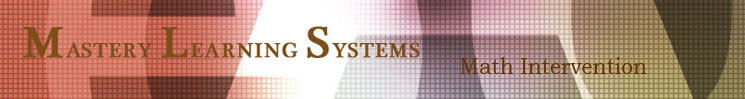K-8 Guided Student Discovery Math for Perimeter, Area, and Volume

Discover the Basics - Perimeter, Area, & Volume
Emailed to you for School, Homeschool, and Tutor

 Order just the lessons you need or the whole book with lessons laid out developmentally, answer keys, and diagnostics. Email orders are placed through PayPal and are processed within 24 hours.Perimeter, Area, & Volume Introduction, 7 Complete Sections (C) The Whole Book (WB)
PAV Introduction (to get the most out of the lessons in this book)
 Helpful math teaching and learning ideas, how the book is laid out, learning perimeter, area, volume, plus a few model lesson guides (8 pages) - \$1.49
Perimeter
 C - What is Perimeter, fluency, find missing dimension, feet, metric (37 pages) - \$6.99
Area
 C - What is Area, fluency, missing area, area and cost, estimate, area within area, area of rectangle, triangle, parallelogram, trapezoid (78 pages) - \$13.49
Circumference of Circles
 C - What is a Ratio, comparing squares, hexagons, circles, circumference/diameter, estimating, +/- square roots, squaring sums and difference (29 pages) - \$4.99
Area of Circles
 C - How to square numbers, squaring the radius, complicated areas, mixed circumference and area problems, mixed areas various shapes (26 pages) - \$4.49
Perimeter of Triangles a2 + b2 = c2
 C - Review of squared numbers, square roots, Pythagoras, 3-4-5 right triangles, perimeter, estimating, hypotenuse on a graph, factoring roots (44 pages) - \$7.49
Surface Area
 C - What is Surface Area, constructing rectangular solids, calculating surface areas (12 pages) - \$2.29
Volume
 C - What is Volume, cubic units, comparing linear, square and cubic units, volume of rectangular solids, with factions and decimals, triangular prisms, cylinders (24 pages) - \$4.29
WB - Discover the Basics - PAV, Teacher - \$45.95
WB - Discover the Basics - PAV, Home - \$29.95Watch our Podcasts on how to use the Graphic Pages and Partner Pages to introduce, reinforce,and extend student work in basic Math Concepts and Procedures.
 Mastery Learning Systems, 532 N. School Street, Ukiah, CA 95482, 800-533-4181, mastery@pacific.net